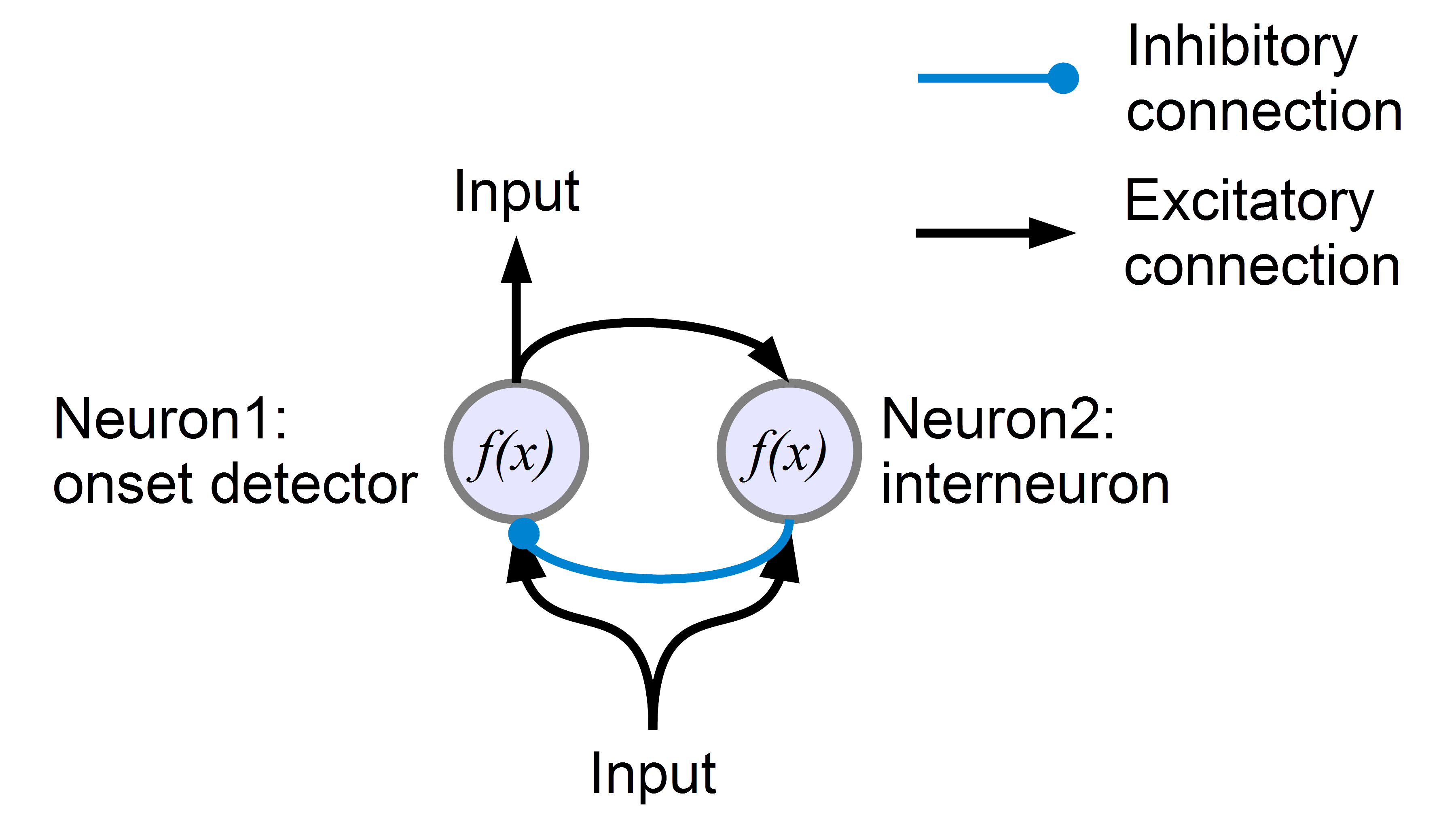# Minimal onset detector and parameter regression

The following code examples represent four distinct codes to solve in different ways the task of generating a small onset detector neural network, as portrayed in the figure below:An arbitrary input reaches two units performing a simple (identical) computation. The output unit (neuron 1) receives the excitatory input and, with a very short delay due to the longer path of the signal, it also receives inhibitory signal from the interneuron (neuron 2). As a result, the output unit exhibits neural activity for a very short time, starting when it receives the outside input stimulus and ending with the inhibitory signal from the interneuron.

In the first example, the parameters required to set the weights of the neural system have been “hand tuned”, therefore have been found by a process of trial and error.

The second example merely proposes a different version of the script generating the network. This is meant to illustrate the use of a function for the computation of each unit/neuron in the network. Due to the presence of the function, this new version of the code requires an extra file (download here format .rar) which is also reported at the end of this page.

For the third and fourth examples, let’s consider an auditory input provided to a volunteer during an experimental task. Imagine the input keeps a certain tone for a few seconds, then increases for another few seconds, before ending. This simple task leads to neural activity in two sensory areas of the cortex: in one case the fMRI has revealed onset detection, in the second case a linear response to the intensity of the stimulus (i.e. the stronger the auditory stimulus, the stronger the BOLD activity). These two BOLD activity recorded via fMRI represent a “target” and we want to find what parameters in the network allow simulating such activity. Both examples use as as input and target the results of the previous simulation (example 2). The final parameters can be interpreted as a simple version of effective connectivity values among areas in the brain, not dissimilar from DCM results.

In the third example the parameters will be searched randomly, running the simulation multiple times with multiple random parameters, and finally selecting the best fit (Montecarlo method).

In the fourth example the parameters will be generated following an heuristic inspired by the process of Darwinism and survival of the fittest: random variations of parameters will be generated in a range, starting from the best known results (Genetic Algorithm method).

### Code: Example 3

Below the code relative to the main file. Download the entire package (3 files): Onset_Montecarlo

### Code: Example 4

Below the code relative to the main file. Download the entire package (3 files): Onset_GeneticAlgorithm

### EXTRA Code: Function named “neural_step” used to simulate neural activity

Insert math as
$${}$$